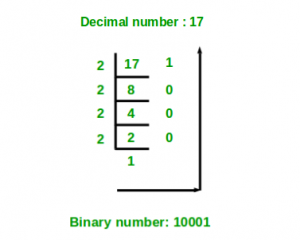# Python program to convert decimal to binary number

• Difficulty Level : Easy
• Last Updated : 28 May, 2022

Given a decimal number as input, the task is to write a Python program to convert the given decimal number into an equivalent binary number.
Examples :

```Input : 7
Output :111

Input :10
Output :1010```

Method #1: Recursive solution```DecimalToBinary(num):
if num >= 1:
DecimalToBinary(num // 2)
print num % 2 ```Below is the implementation of the above recursive solution:

## Python3

 `# Function to convert decimal number` `# to binary using recursion` `def` `DecimalToBinary(num):` `    `  `    ``if` `num >``=` `1``:` `        ``DecimalToBinary(num ``/``/` `2``)` `    ``print``(num ``%` `2``, end ``=` `'')`   `# Driver Code` `if` `__name__ ``=``=` `'__main__'``:` `    `  `    ``# decimal value` `    ``dec_val ``=` `24` `    `  `    ``# Calling function` `    ``DecimalToBinary(dec_val)`

Output

`011000`

Method #2: Decimal to binary using in-built function

## Python3

 `# Python program to convert decimal to binary` `  `  `# Function to convert Decimal number ` `# to Binary number ` `def` `decimalToBinary(n): ` `    ``return` `bin``(n).replace(``"0b"``, "") ` `  `  `# Driver code ` `if` `__name__ ``=``=` `'__main__'``: ` `    ``print``(decimalToBinary(``8``)) ` `    ``print``(decimalToBinary(``18``)) ` `    ``print``(decimalToBinary(``7``)) `

Output

```1000
10010
111
```

Method #3:Without in-built function

## Python3

 `# Python program to convert decimal to binary` `  `  `# Function to convert Decimal number ` `# to Binary number ` `def` `decimalToBinary(n): ` `    ``return` `"{0:b}"``.``format``(``int``(n))` `  `  `# Driver code ` `if` `__name__ ``=``=` `'__main__'``: ` `    ``print``(decimalToBinary(``8``)) ` `    ``print``(decimalToBinary(``18``)) ` `    ``print``(decimalToBinary(``7``)) `

Output

```1000
10010
111
```

Quick Ninja Method: One Line Code to Convert Decimal to Binary with user input

## Python3

 `# Quick Ninja One line Code` `print``(``bin``(``4785``)[``2``:])`

Output

```1001010110001
```

or

## Python3

 `# Use this for user input` `#decNum = int(input("Enter any Decimal Number: "))`   `decNum ``=` `4785` `print``(``bin``(decNum)[``2``:])`   `decNum1 ``=` `10` `print``(``bin``(decNum1)[``2``:])`   `decNum2 ``=` `345` `print``(``bin``(decNum2)[``2``:])`

Output

```1001010110001
1010
101011001
```

My Personal Notes arrow_drop_up
Recommended Articles
Page :